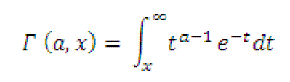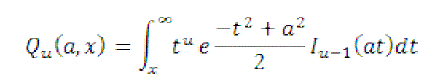ISSN ONLINE(2320-9801) PRINT (2320-9798)

All submissions of the EM system will be redirected to Online Manuscript Submission System. Authors are requested to submit articles directly to Online Manuscript Submission System of respective journal.

# Energy Efficient Cognitive Radio Hybrid Voting Rule Â–PSO

 Parth Gulati1, Dr. Rajesh Khanna2 M.E(scholar), Department of Electronics & Communication Engineering, Thapar University, Patiala, India Professor, Department of Electronics & Communication Engineering, Thapar University, Patiala, India Related article at Pubmed, Scholar Google

Visit for more related articles at International Journal of Innovative Research in Computer and Communication Engineering

## Abstract

In this paper, a cooperative framework in cognitive radio networks is proposed. A Cognitive radio is an extension to SDR, where one allows the radio system to adapt to its environment through learning or artificial intelligence with the aim of increasing performance. Bit error rate and signal to noise ratio are used to check the efficiency. BER is defined by the number of inaccuracies per reassigned data for the time interval during which channel is active and signal to noise ratio is the power ratio between a signal (meaningful information) and the background noise (unwanted signal). In this research PSO techniques are used by which the results are optimized.

### Keywords

BER, Cognitive Radio, PSO, SNR, Cooperative Spectrum Sensing, Optimization

### SPECTRUM SENSING

A CR network collected of K CRs (unauthorized users) and a common recipient is consideedr. Each CR perform spectrum sensing separately and after that the local result are sent to the frequent receiver which can fuse all existing result in order to gather the absence or presence of the authorized user. The real meaning of spectrum sensing is a binary hypothesis-testing problem.
H0: Primary user is not present;
H1: Primary user is present.
In the following we the spectrum sensing at CR ?? is considered. The sensing method is to choose between the following two hypotheses:Where xi(t) is the received signal at the ith CR in time slot t,s(t) is the PU signal, Wi(t) is the preservative white Gaussian noise (AWGN), and hi(t) denotes the compound channel gain of the sense guide between the authorized and the ith CR. The sensing time is lesser than the coherence time of the channel. Then, the sensing channel hi(t)can be view as time-invariant during the sensing process. Without loss of generality, hi(t),is denoted as hi.The position of the PU remains unaffected during the spectrum sensing process. If prior information of the authorized signal is unknown, the method energy detection is most constructive for detecting zero-mean constellation signals . For the ith CR with the energy detector, the average possibility of false alarm, the average possibility of detection, and the average possibility of missed detection over AWGN channels are given, respectively, byIn the above equations, λi signify the energy recognition threshold and the immediate signal-to-noise ratio (SNR) at the ith CR, respectively,γi is the time-bandwidth creation of the energy detector, Γ (a,x) is the incomplete gamma function given byΓais the gamma function, and Qu(a,b) is the universal Marcum Q function given bywithIu-1(.) being the adapted Bessel function of the first kind and order u– 1. In cooperative spectrum sensing, each cooperative partner makes a binary choice based on its incomplete study and then ahead one bit of the decisionDi(1 standing for the presence of the PU, 0 for the lack of the PU) to the common receiver through an error-free channel. At the ordinary receiver, all 1-bit decisions are multipart together according to logic ruleWhere and denote the inference drawn by the general receiver that the PU signal is not transmitted. The threshold n is an integer, representing the “n out of K” voting rule. It can be seen that the OR rule corresponds to the case of n=1 and the AND rule correspond to the case of n=K. In the case of an AWGN channel, is free of .In the case of a Rayleigh fading channel, let be averaged over the figures of . For both kinds of channels, = 1−. So, the false alarm probability of cooperative spectrum sensing is given byThe missed detection probability of cooperative spectrum sensing is given by### SYSTEM MODEL

This section presents the details of the cooperative cognitive radio networking model under consideration, together with the main system parameters
A. MAC layer
As shown in Fig. 1, there are two kinds of networks, the infrastructure-based primary network and ad hoc secondary network, collocated in the same area. In the primary network, the PUs communicates with the base station (BS) in time division multiple access (TDMA) mode. The time slot duration for each PU’s transmission is T. In secondary network, the SU transmits data to its corresponding receiver. The PU can select one SU for cooperation and the Amplify-and-Forward (AF) cooperative mode is considered. The PU grants the use of the bandwidth to the cooperative SU so as to improve the energy efficiency of the communication to the BS.
B. Physical layer
The channels between nodes can be modeled as independent proper complex Gaussian random variables, constant within each slot, but generally varying over the slots. We use the following notations to denote the instantaneous channels in each slot.
C. Security threats
The PU selects an SU for cooperation from multiple candidates according to the utility. The SU, with whom the PU can gain the highest utility through cooperation, will be chosen. If all the SUs are well-behaved, both PU and SU can benefit from the cooperation. However, when there exist some dishonest or malicious SUs, the normal operation of CCRN will not be guaranteed. Specifically, the following possible security issues arising in CCRN need to be addressed. In CCRN, the data being transmitted from the PU can be eavesdropped by the neighboring SUs such that the confidentiality cannot be guaranteed.

### PSO ALGORITHM

The particle swarm optimization (PSO) ,  is a mathematical technique that augments a delinquent by repeatedly trying to mend a contender clarification in consideration to a prearranged ration of eminence. PSO boosts a problem by partaking a lot of contender elucidations, which are taken as christened specks in this, and affecting these units around in the searchspace in agreement to simple scientific formulae above the particle's position and velocity. Each particle's association is opinionated by its local premium known site and it's also guide on the way to the premium known positions in the searchspace, which are reorganized as recovered positions are start by other particles.
This is projected to move the swarm toward the finest option. Particle swarm optimization algorithm is originally credited to Kennedy, Eberhart and Shi . It was first proposed for simulating social actions, as a conform representation of the movement of organisms in a bird group or fish school. The algorithm was simplified and it was pragmatic to assess optimization. The book by Kennedy and Eberhart describes many thoughtful aspects of Particle Swarm Optimization and swarm intelligence. An broad investigation of PSO applications is made by Poli and which gave the idea to use PSO for different applications in different fields of engineering. The PSO is a meta heuristic so it makes few or no assumption about the difficulty being optimized and can search very large spaces of aspirant solutions. However, meta heuristics such as PSO do not agreement an optimal result is ever found. In particular, PSO does not use the ascent of the problem being optimized, which means PSO does not require that the optimization difficulty be differentiable as is requisite by classic optimization methods such as gradient fall and quasi-Newton methods. These are some disadvantages of PSO algorithm in contrast to other. But there are some special features that are PSO can also be used on optimization problems that are incompletely irregular, noisy, change over time, etc.

### BIT ERROR RATE (BER)

In communication system the bit error rate is defined as the ratio of number of error bits and total number of bits transmitted during a precise period. In digital transmission or digital communication system, the number of bit error is the number of recognized bits of a data stream over communication channels that have been changed due to noise, obstruction, distortion or bit synchronization errors in the system. The bit error rate or bit error ratio (BER) is the number of bit error divided by the total number of shift bits during a considered time period. BER is a unit less performance measure; often expressed as a percentage (%).While the basic concept of BER measurement is simple send a data stream through the system and compare the output to the input its execution is not trivial.
In communication system the bit error rate is defined as the ratio of number of error bits and total number of bits transmitted during a precise period. In digital transmission or digital communication system, the number of bit error is the number of recognized bits of a data stream over communication channels that have been changed due to noise, obstruction, distortion or bit synchronization errors in the system. The bit error rate or bit error ratio (BER) is the number of bit error divided by the total number of shift bits during a considered time period. BER is a unit less performance measure; often expressed as a percentage (%).While the basic concept of BER measurement is simple send a data stream through the system and compare the output to the input its execution is not trivial.

### SIGNAL TO NOISE RATIO

Signal to noise ratio (often abbreviated SNR or S/N) is a measure used in discipline and engineering to quantify how much a signal has been penniless by noise. It is defined as the fraction of signal power to the noise power affecting the signal. A ratio higher than 1:1 indicate further signal than noise. While SNR is commonly quoted for electrical signals, it can be applied to any form of signal (such as isotope levels in an ice core or biochemical siganling between cells). Signal-to-noise ratio" is sometimes used informally to refer to the ratio of useful information to false or inappropriate data in a conversation or exchange. For example, in online discussions forums and other online communities, off topic posts and spamare regarded as "noise" that interfere with the "signal" of proper discussion.

### RESULTS AND CONCLUSION

The work proceed possesses the following results of comparison using PSO and without using PSO.
CRs in AWGN with SNR = 10 dB. The above figure represents a comparative result of total error rate with and without using PSO. It is found that the difference in the result is of .3 units in error i.e the PSO has minimized the error by .3 units.
Figure 5: Total error rate of cooperative spectrum sensing versus number of collaborating cognitive radios in a network with 50 CRs in Rayleigh fading with SNR = 5, 10, 15, 20 dB with and without PSO The above figure represents the comparison with and without using pso at different levels like 5 db ,10 db ,15 db and results are found to be optimized by .2 to .45 units.
Figure 6: Optimal voting rule (versus detection threshold) of cooperative spectrum sensing in AWGN channel with PSO and without PSO and SNR = 0, 5, 10 dB The above figure represents the optimal voting rule and according to it if the optimal voting rule is found in a lesser node the optimization is successful. From the above figure it is quite clear that without using PSO and with PSO has one unit difference.
Cognitive radio allows unauthorized users to access authorized frequency bands through active spectrum use so as to reduce band shortage. It require intellectual band sensing techniques like co-operative sensing which makes use of information from number of user. In this paper, PSO algorithm is used that decreases the bit error rate. Efficiency increases with the increase of Signal to Noise Ratio.

### Figures at a glanceFigure 1 Figure 2 Figure 3Figure 4 Figure 5 Figure 6